# Graphing a Linear Inequality on the Number Line Online Quiz

#### Better Business Writing Skills

13 Lectures 1.5 hours

#### Freelance Writing 101: Start Your Successful Writing Career

25 Lectures 1 hours

#### Writing That Stands Apart

14 Lectures 1 hours

Following quiz provides Multiple Choice Questions (MCQs) related to Graphing a Linear Inequality on the Number Line. You will have to read all the given answers and click over the correct answer. If you are not sure about the answer then you can check the answer using Show Answer button. You can use Next Quiz button to check new set of questions in the quiz.### Explanation

Step 1:

We first locate the point 27 on the number line.

Step 2:

We put a open circle on 27 and draw a thick line towards right to denote the inequality x > 27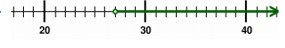### Explanation

Step 1:

We first locate the point 14 on the number line.

Step 2:

We put an open circle on 14 and draw a thick line towards left to denote the inequality x < 14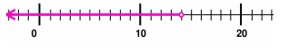### Explanation

Step 1:

We first locate the point −42 on the number line.

Step 2:

We put a closed circle on −42 and draw a thick line towards left to denote the inequality x ≤ −42### Explanation

Step 1:

We first locate the point −32 on the number line.

Step 2:

We put a open circle on −32 and draw a thick line towards right to denote the inequality x > −32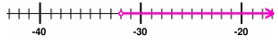### Explanation

Step 1:

x ≥ 5

We first locate the point 5 on the number line.

Step 2:

We put a closed circle on 5 and draw a thick line towards right to denote the inequality x ≥ 5### Explanation

Step 1:

x – 2 ≥ 6; x – 2 + 2 ≥ 6 + 2; x ≥ 8

We first locate the point 8 on the number line.

Step 2:

We put a closed circle on 8 and draw a thick line towards right to denote the inequality x ≥ 8### Explanation

Step 1:

x – 3 ≤ 10; x – 3 + 3 ≤ 10 + 3; x ≤ 13

We first locate the point 13 on the number line.

Step 2:

We put a closed circle on 13 and draw a thick line towards left to denote the inequality x ≤ 13### Explanation

Step 1:

2x < 18; x < $\frac{18}{2}$; x < 9

We first locate the point 9 on the number line.

Step 2:

We put an open circle on 9 and draw a thick line towards left to denote the inequality x < 9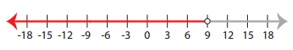### Explanation

Step 1:

$\frac{x}{7}$ ≤ 3; x ≤ (7 × 3); x ≤ 21

We first locate the point 21 on the number line.

Step 2:

We put a closed circle on 21 and draw a thick line towards left to denote the inequality x ≤ 21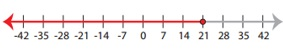### Explanation

Step 1:

x + 2 > 8; x > 8 – 2; x > 6

We first locate the point 6 on the number line.

Step 2:

We put an open circle on 6 and draw a thick line towards right to denote the inequality x > 6graphing_linear_inequality_on_number_line.htm